# Introduction to Absorption

Absorption of electromagnetic radiation (light) occurs when the energy of a photon is transferred to matter. Absorption can occur in atoms by promoting an electron to higher energetic states. In molecules, absorption can result from transitions into higher electronic, vibrational, and rotational states. The absorption of light is fundamental process relevant to many photonics materials and devices.

## Plank's relation

Light can be characterized in multiple ways, notably, either by its frequency, which is the number of oscillations per second, or its wavelength, literally the distance between peaks in a sinosoidal curve. Also, for any wave, the product of its wavelength times its frequency is the speed of that wave. For example, the speed of light traveling in a vacuum is roughly 3x 10^08 m/s.

In the early 20th century, Planck showed that the energy of a photon can be related to its frequency by using the Planck relation (also called the Planck-Einstein equation)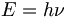$E=h \nu\!$

or, since λν = c: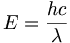$E=\frac {h c}{\lambda} \,\!$

where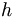$h\,\!$ is Planck's Constant$\nu\,\!$ is frequency$\lambda\,\!$ is wavelength

Thus, the energy of the light is directly proportional to its frequency and inversely proportional to its wavelength. One distinction that is important to understand is that the energy of light as a function of a frequency and also the field strength of light . The strength of the field of light is called the intensity of light. If it is very intense and you look into it, it can blind you. The energy we are discussing here is the energy of a photon. If there is a ground state and an excited state, there will be a certain wavelength of light that is required in order to achieve an absorption by the molecule.

## Excitation vs polarization

A molecule can interact with light and light can be absorbed. There is a transition with a starting state and a final state. These lines the HOMO of the molecule to the LUMO of the molecule. A beam of light excites the molecule causing one electron to jump from the HOMO (a filled orbital) to the LUMO (an unfilled orbital). This is a simple one electron picture. If there is a true absorption of a photon, this state will have a finite lifetime. That means that after the electric field is removed, (i.e the light is shut off,) the molecule will be metastable in that state and will come back down to the ground state with a certain characteristic lifetime. Even if the light does not have enough energy to promote an electron from one level to another, it can still get the electrons in the molecule to resonate back and forth and oscillate; this process is referred to as polarization. However, as soon as that field is removed, the molecule will be instantaneously back in its ground state.

In the case of excitation / absorption the energy has transferred from the electric field to the system and it is stored temporarily, or in other words, gained potential energy. That potential energy is associated with this electron not being in the ground state. In the case of polarization, no energy is stored in the molecule. Therefore, two situations can occur; in one case there is a transference of energy to the molecule, and in the other case, there isn’t. There are several parameters that can be used to characterize the transition of the promotion of the electron. One of the parameters is the energy of the photon and the associated wavelength that is required to promote the electron from one level, to the one above it. Another parameter is the inherent ability of a molecule to absorb the light. The presence of two states, one below and above, and the addition of a photon that has the appropriate energy to couple those two states and allow the electron to be promoted from the lower state to the higher state energetically, is not sufficient to assure that light will be absorbed. There are other factors about the molecule that have to be met in order for the molecule to absorb the light beam. This is illustrated by absorption spectra; some absorption bands are strong and some are weak. This means that certain transitions are more allowed than others and certain transitions are forbidden, which is because they are not quantum mechanically allowed.

## Solvent effects

The Planck relation tells us exactly what energy is required of the photon to get the promotion of an electron from a ground state to an excited state.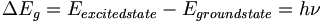$\Delta E_g = E_{excited state} - E_{ground state} = h \nu\,\!$

Basically, the energy of the photon should equal to the difference in energy of the excited state and the energy of the ground state(excited state energy – ground state energy). A photon of frequency ν corresponds to the energy gap between states. In real molecules, it is not always true that there is only a single energy able to excite the molecule. Molecules have a variety of vibrational states and rotational states. Also, molecules as at least in the condensed state as in a polymer or in solution, are fundamentally inhomogeneous. When a molecule is put into a solvent, it is very unlikely that there will be another molecule in the solution that has exactly the same orientation of every solvent molecule around it or in the 2nd sphere around it that will be identical. Consequently, for each molecule that has a different solvation sphere will have slightly different energy. It could be too small to measure but in some cases, it can be quite dramatic.

The question now is “what would give rise to different energies?”. If you take a polar molecule and put it into a polar solvent. The dipoles of the solvent will reorient around the ground state dipole of the molecule in such a way to stabilize it. This will lead specifically to an energy stabilization of the system. Now all of those solvent molecules are not static so in any given time, the molecule is actually surrounded by solvent molecules that are moving. Since there are many different solvent molecules, each molecule is going to have a slightly different orientation of the solvent around. This is going to cause the slightly different stabilization of the ground state. It turns out that the excited state of the molecule can have a dipole moment and the excited state dipole need not be the same as the ground state dipole. In addition, the excited state might be stabilized differently than the ground state. All these factors can lead to further broadening within the same solvent. So to draw an absorption spectra as one frequency is a completely idealized case because molecules themselves have vibrational states and broadening of their spectra due to the fact that molecules can be in different solvents. There are also quantum mechanical aspects that lead to uncertainty in measurement of that energy.

## Energy Units

There are different units of energy used to characterize photon energies and all energies. This is very important to understand because it helps you move along between the language of physics, language of chemistry, and the language of spectroscopy. The units of energy are: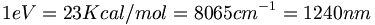$1 eV = 23 Kcal/mol = 8065 cm^{-1}= 1240 nm\,\!$

This is worth memorizing.